# Financial Engineering (I) #2 – Introduction to No ArbitrageConsider the following contract
1. Pay the price p at t=0.
2. Receiveat time t=k, k = 1, …, T
*Cash flowcan be negative

So what does the above contract mean? One pays a price p at time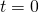in order to receive this contract and get this cash flow. Thus at, and all the way up to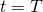, we get cash flow. So we want to introduce the ideas of no arbitrage to set price p.

The no-arbitrage condition bounds the price p for this contract.
(i) Weak No-Arbitrage: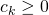for all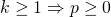(ii) Strong No-Arbitrage: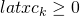for all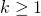andfor someThis conditions both essentially eliminates the possibility of a free lunch!

Here is a intuitive rationale for the weak no-arbitrage condition: Suppose. Sincefor all, the buyer receives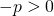at time 0 and then does not lose money thereafter. This will lead to free lunch! Seller can increase price price as long as, still have buyers available. Essentially, buyers will be willing to pay a higher price in order to compete.

Lets look at the rationale for the strong no-arbitrage condition. Suppose. Sincefor some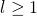, we have a free lunch as long asBoth of these conditions are motivated by the fact that in a market, if there are contracts which you get something for nothing, then just by Law of Supply and Demand, the contract will be priced to a point where you must pay a fair price.

Under the no-arbitrage condition, the following are implicit assumptions we make.
1. Markets are liquid, i.e., we have sufficient number of buyers and sellers
2. Price information is available to all buyers and sellers
3. Competition in supply and demand will correct any deviation from no-arbitrage prices.

Suppose one is able to borrow and lend unlimited amounts at an interest rate of r per year, how to we find the price p of a contract that payslatex \frac{A}{1+r}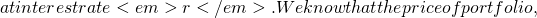latex z = p – \frac{A}{1+r}latex A – A = 0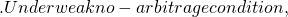latex c_1 \ge 0 \Rightarrow z \ge 0 \Rightarrow p \ge \frac{A}{1+r}latex \frac{A}{1+r}latex z = \frac{A}{1+r} – platex -A + A = 0latex c_1 \ge 0 \Rightarrowlatex z \ge 0 \Rightarrow p \le \frac{A}{1+r}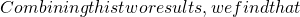latex p = \frac{A}{1+r}\$.

However, the above results is possible as we assume we can borrow and lend at rate r. Thing will be different is borrowing and lending rates are different, or if the borrowing and lending markets are elastic.

Not readable? Change text.